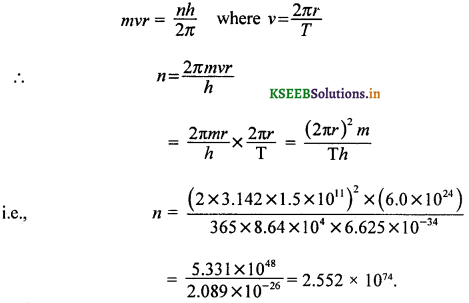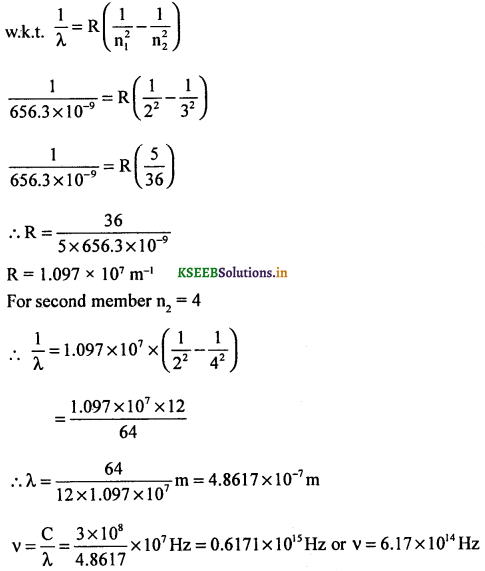# 2nd PUC Physics Question Bank Chapter 12 Atoms

Students can Download 2nd PUC Physics Chapter 12 Atoms Questions and Answers, Notes Pdf, 2nd PUC Physics Question Bank with Answers helps you to revise the complete Karnataka State Board Syllabus and to clear all their doubts, score well in final exams.

## Karnataka 2nd PUC Physics Question Bank Chapter 12 Atoms

### 2nd PUC Physics Atoms One Marks Questions and Answers

Question 1.
Who discovered electrons?
Electrons were discovered by J.J. Thomson in the year 1897.

Question 2.
What is the electrical charge on an atom?
At atom of an element is electrically neutral.Question 3.
Who proposed the first model of an atom?
J.J. Thomson proposed the first model of an atom in the year 1898.

Question 4.
What is plum pudding model?
According to this model, the positive charge is distributed throughout the volume of the atom and negatively charged electrons are embedded in it like plums in the pudding, (also like seeds in a watermelon).

Question 5.
Electromagnetic radiations are produced due to what effect?
Electromagnetic radiations are due to oscillations of atoms and molecules governed by the interaction of each atom or molecule with its neighbours.

Question 6.
Give any one source which emits electromagnetic radiations forming a continuous emission spectrum of several wavelengths differing in intensities.
Condensed matter like solids and liquids and non – condensed matter like dense gases at all temperatures emit e.m. radiations of several wavelengths as a continuous spectrum.

Question 7.
As per which model do atoms become unstable?
Rutherford’s atom model. An accelerating electron radiates energy and spirals around the nucleus. Ultimately electrons should fall inside the nucleus. Hence Rutherford model does not explain stability of the atom.

Question 8.
What is a stationary orbit?
A stationary orbit is one in which the revolving electron does not radiate energy.

Question 9.
Give the relation between radius and principal Quantum number of an atom.
rn ∝ n2.

Question 10.
Are the electron orbits equally spaced?
Electron orbits are unequally spaced.

Question 11.
What is the relation between the energy of an electron and the principle Quantum, number?
En ∝ $$\frac{1}{n^{2}}$$Question 12.
What is an excited state of an atom?
When the atom is given sufficient energy, the transition takes place to an orbit of higher energy. The atom is then said to be in an excited state.

Question 13.
What is meant by the wave number of spectral line?
Wave number represents number of waves present in one metre length of the medium.

Question 14.
What is the value of Rydberg’s constant?
R = 1.097 × 107 m-1.

Question 15.
What is ionization energy?
The energy required to shift an electron from its original orbit to an infinite orbit distance away from the nucleus is called ionization energy. It is expressed in eV.

Question 16.
What is excitation energy?
The energy required for shifting an electron from its orbit to a higher orbit is called excitation energy. It is expressed in eV.

Question 17.
Define electron volt.
Electron volt is defined as the energy gained by an electron in falling through a potential difference of IV.

### 2nd PUC Physics Atoms Two Marks Questions and Answers

Question 1.
How does the spectrum emitted by rarefied gases differ from those of dense gases?
In rarefied gases, the separation between atoms or molecules are farther apart. Hence the atoms give discrete wavelengths without any interaction with the neighbouring atoms, e.g.,

• rarefied gases heated in a flame,
• rarefied gases excited electrically.

Question 2.
Give any one difference between Thomson’s model and Rutherford’s model of an atom.
In the Thomson’s atom model, electrons are in stable equilibrium while in the Rutherford’s atom model electrons always experience a net force $$\frac{m v^{2}}{r}$$ due to electrostatic force of attraction between electron and nucleus.Question 3.
Write the expression for the radius of nth orbit of the hydrogenic atoms and give the meanings of the symbols used.
r = $$\frac{\varepsilon_{0} n^{2} h^{2}}{\pi m Z e^{2}}$$, where V is the radius of the orbit, n is the principal quantum number, h is the Planck’s constant, m is the mass of an electron, Z its atomic number and e is the quantised unit of charge and e0 absolute permittivity for air.

Question 4.
Give the expression for velocity of an electron in the nth orbit. Explain the meanings
v = $$\frac{Z e^{2}}{2 \varepsilon_{0} n h}$$, where Z is the atomic number, e is the quantised unit of charge, n is the principle quantum number, h is the Planck constant and E0 is absolute permitivity of the free space.

Question 5.
Write the formula for the wave number of a spectral line.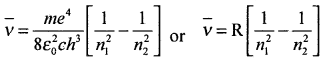where, R is Rydberg’s constant, R = 1.097 × 107 m-1, n1 and n2 are energy levels.

Question 6.
What is the expression for the Rydberg’s constant? Give the meaning of the symbols.
R = $$\frac{m e^{4}}{8 \varepsilon_{0}^{2} c h^{3}}$$ where
m – mass of the electron,
e – charge on the electrons,
c – speed of light, and
h – Planck’s constant and
ε0 – absolute permittivity of the free space.

Question 7.
Write the formula for the wave number of the spectral lines of Lyman series.
Lyman series consists of spectral lines corresponding to the transition of an electron from higher energy orbits to the first orbit, i.e., n = 1 and n2 = 3, 4, 5 ……. ∞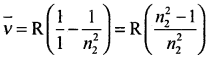Question 8.
Write the formula for the wave number of the spectral lines of Balmer series.
Balmer series consists of spectral lines emitted during transitions of electron from higher energy orbits to the second orbit, i.e., n1 = 2 and n2 = 3, 4, 5, ……. ∞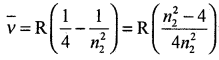Question 9.
Mention any two merits of Bohr’s atom model.

• The atom model provided the basic foundation for the development of quantum mechanics.
• The value of Rydberg’s constant R was arrived theoretically.

Note: Other merits are:

• The theory for the first time predicted the size of an atom in terms of absolute constants.
• This model made use of the principle of quantisation of angular momentum and energy states of hydrogen and hydrogenic atoms.

Question 10.
Mention any three demerits of Bohr’s atom model. (March 2014)
Ans:

1. The theory is applicable only for the hydrogen atom.
2. The relativistic variation of mass is not taken into account in the theory.
3. The fine structure of spectral lines could not be explained.
4. This theory or atom model fails to explain the relative intensities of spectral lines.

Question 11.
How does Rydberg’s constant vary with atomic number?
R = Z2RH, where Z = atomic number.
RH = Rydberg’s constant for hydrogen atom.
Hence, R ∝ Z2 for hydrogenic atoms.

Question 12.
What is the value of ionization potential of <sup.42He atom?
I. E = -(13.6eV)Z2
Put Z = 2.
∴ I.E = – 13.6 (2)2 eV = – 54.4 eV.
∴ Ionisation potential = – 54.4 V.

Question 13.
Name the physicists who for the first time verified the wave nature of electrons.
C.J, Davisson and L.H. Germer verified the wave nature of electrons.

Question 14.
Name the two quantised conditions proposed by Bohr in the atom model.
Bohr proposed

• quantisation of energy states related to the transition of electrons from one orbit to another,
• quantisation of orbit or angular momentum.

Question 15.
Write the mathematical conditions for quantisation of orbits and energy states.

• mvr = $$\frac{n h}{2 \pi}$$ → quantisation of angular momentum
• E2 – E1 → qunatisation of energy states and resulting transition

Question 16.
What are hydrogenic atoms?
Hydrogenic (Hydrogen like) atoms are the atoms consisting of a nucleus with positive charge ‘+Ze’ and a single electron. Here ‘Z’ is atomic mass number and ‘e’ is the quantised unit of charge.
eg., singly ionised helium, doubly ionised lithium:### 2nd PUC Physics Atoms Three Marks Questions and Answers

Question 1.
Write de-Broglie wavelength associated with 3r

d and 4th orbit in Bohr’s atom model.
According to quantisation rule of Bohr’s model,Question 2.
Give de-Broglie’s explanation of quantisation of angular momentum as proposed by Bohr.
The condition for stationary wave formation is that the total distance travelled between the nodes(two) up and down or given path is integral multiple of ‘λ’
i.e., 2πrn = nλ where n = 1,2,3, …..Question 3.
Relate KE, PE and TE (total energy) of an electron of hydrogenic atoms.
1. Potential Energy = 2 times the total energy.
2. Kinetic Energy of an electron = – TE
where, total energy is negative and E = $$-\frac{Z^{2} m e^{4}}{8 \varepsilon_{0}^{2} n^{2} h^{2}}$$
For H2 atom, Z = I
For H2 like atoms, charge on the nucleus = +Ze.Question 4.
How is the frequency of radiation different from that of frequency of electron in its orbit?
(i) For radiation frequency v = $$\frac{\mathrm{E}_{2}-\mathrm{E}_{1}}{h}$$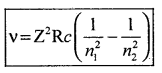where n2 = higher orbit and n1 = lower orbit.
(ii) For frequency of electron revolution in its orbit, f = $$\frac{1}{T}=\frac{v}{2 \pi r}$$

Question 5.
Arrive at the expression for electric current established due to the motion of electron in its orbit.
By definition, electric current i = $$\frac{q}{\mathrm{T}}$$
where, q = e and T = $$\frac{2 \pi r}{v}$$
∴ i = $$\left(\frac{e v}{2 \pi r}\right)$$ampere.

Question 6.
Arrive at the expression for the magnetic field at the centre of the circular path due to an orbiting electron.
We know that the magnetic field at the centre = $$\frac{\mu_{0}}{4 \pi} \cdot \frac{2 \pi i}{r}$$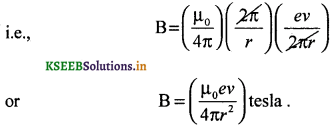Question 7.
Arrive at the expression for the magnetic flux density at the centre of the circular path of an electron in terms of magnetic moment.
We know that
B = $$\left(\frac{\mu_{0}}{4 \pi}\right)\left(\frac{2 \pi}{r}\right) i$$
Multiplying and dividing by r2 we get,
B = $$\left(\frac{\mu_{0}}{2 r}\right)\left(\frac{i \pi r^{2}}{\pi r^{2}}\right)$$where, m = i(πr2) is known as the magnetic moment associated with the current loop.

Question 8.
Why do we use gold in Rutherford’s α – particle scattering experiment?
The reasons are

• Gold is malleable
• Gold nucleus is heavy and can produce large deflections of α – particles### 2nd PUC Physics Atoms Five Marks Questions and Answers

Question 1.
State the three postulates of Bohr’s theory of hydrogen atom. (March 2014)
1. An electron can not revolve round the nucleus in any arbitrary orbit. Only certain orbits are permitted. Electron does not radiate energy in stationary orbits.

2. The radius of the allowed electron orbits is determined by the quantum condition. It states that the orbital angular momentum of electron about the nucleus is an integral multiple of $$\frac{h}{2 \pi}$$. According to Bohr’s postulate, mvr = $$n\left(\frac{h}{2 \pi}\right)$$

3. An atom radiates energy only when an electron jumps from a higher energy orbit to a lower energy orbit. If E1 and E2 are the lower and higher energies and v is the frequency of radiation, then v = $$\frac{E_{2}-E_{1}}{h}$$.

Question 2.
Derive an expression for the radius of nth Bohr’s orbit of hydrogen atom and hence write the expression for the radius of first orbit of hydrogen atom. (March 2015)
Consider an atom of atomic number Z. The charge on its nucleus is + Ze. Let an electron of mass m and charge ‘-e’ revolve round the nucleus in a circular orbit.
Let v be its velocity.
The coulomb’s electrostatic force of attraction between the electron and the nucleus is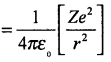This provides the centripetal force $$\frac{m v^{2}}{r}$$ needed for the orbital motion of the electron.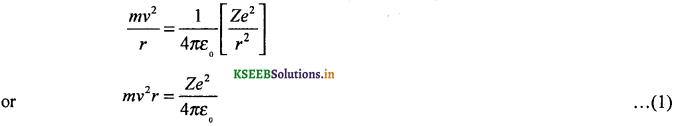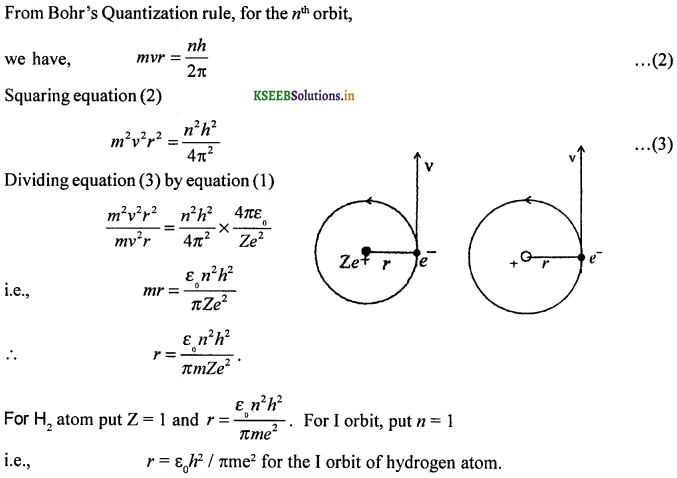Question 3.
Obtain an expression for the total energy of an electron in the nth orbit of hydrogen atom in terms of the radius? (July 2014)
Consider an electron of mass m and charge -e revolving round the nucleus of an atom of atomic number Z in the nth orbit of radius ‘r’. Let v be the velocity of the electron. The electron possesses potential energy, because, it is in the electrostatic field of the nucleus. The electron also possesses kinetic energy by virtue of its motion.
Potential energy of the electron is given by,
Ep = (potential at a distance r from the nucleus) (-e)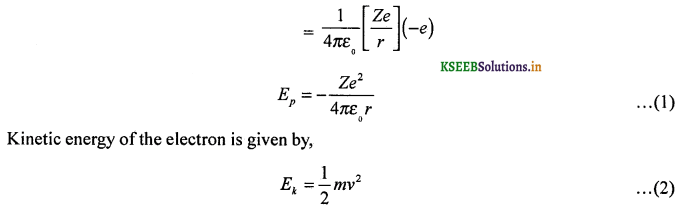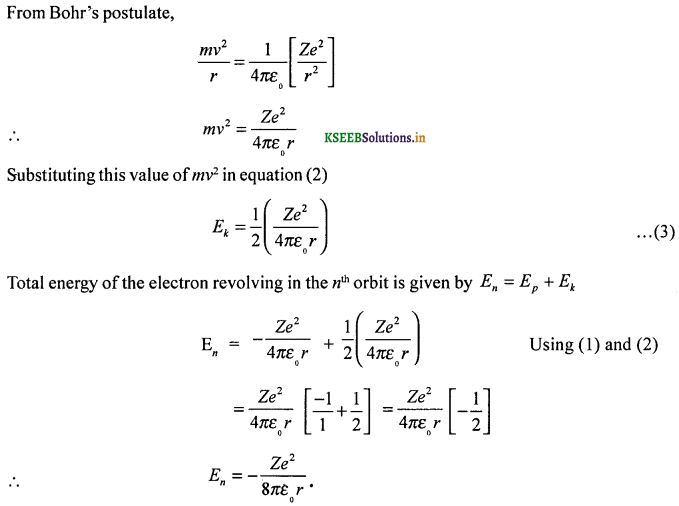Question 4.
Obtain an expression for the total energy of an electron in the nth orbit of hydrogen atom in terms of absolute constants.Question 5.
Give an account of the spectral series of an hydrogen atom.
Hydrogen atom has a single electron. Its spectrum consists of a series of spectral lines.
Lyman series: Lyman Series consists of spectral lines corresponding to the transition of an electron from higher energy orbits to the first orbit n = 1 and n2 = 2, 3, 4 …. ∞
These lines belong to Ultraviolet region.

Balmer series: Balmer series consists of spectral lines emitted during transitions of electrons
from higher energy orbits to the second orbit, n1 = 2, n2 = 3, 4, 5 ….. ∞
These lines lie is the visible region

Paschen series: Paschen series consists of spectral lines emitted when electrons jump from
higher energy orbits to the third orbit n1 = 3, n2 = 4, 5, 6 ……… ∞
These lines lie the in infrared region.

Brackett Series: Brackett series consists of spectral lines emitted during transitions of electrons from higher energy orbits to the fourth orbit.
n1 = 4, n2 = 5, 6, 7 …. ∞
These lines lie in the infrared region.

Pfund Series: P fund series consists spectral lines emitted during transition of electrons from higher energy orbits to the fifth orbit.
n1 = 5 and n2 = 6, 7, 8 ….. ∞
These lines lie in infrared region.

In general the transition from (n1 + 1) to n1 corresponds to first the member or the longest wavelength of any series. The transition from ∞ (infinity) state to ‘n1’ state corresponds to the last number or series limit or shortest wavelength of any series.

Question 6.
Draw the energy level diagram of hydrogen atom. Calculate the energy values upto fifth excited energy states of hydrogen.
The total energy of an electron in its Orbit for an hydrogen atom E = $$\frac{-13.6}{n^{2}} e V$$
Where ‘n’ is known as the principal quantum number whose value n = 1,2,3,……….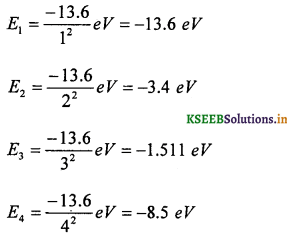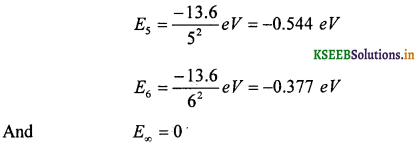The energy level diagram represents different energy states with an increasing energy.
The transition of electrons from higher energy states to lower energy states results in various levels of radiations classified into invisible ultra violet, visible light and invisible infrared spectrum of radiation as illustrated below. –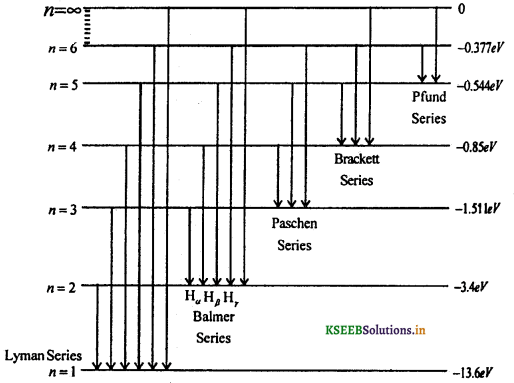Note:
Excitation potential: The amount of energy in electron volt required to excite an electron in a given state to the next higher state is known as the excitation energy. The numerical value of excitation energy expressed in volts is known as the excitation potential.

Ionisation potential: The amount of energy in electron volt required to remove an electron in its ground state to infinity state is known as the ionisation potential. The numerical value of ionisation energy expressed in volts is known as the ionisation potential.Question 7.
Derive an expression for the frequency of spectral series by assuming the expression for the total energy of the electron of hydrogen.
When an atom in the excited state returns to the normal state, the transition results in the radiation of energy,Question 8.
Outline the expeimental study and the condusions arrived in the α – scattering by a gold foil.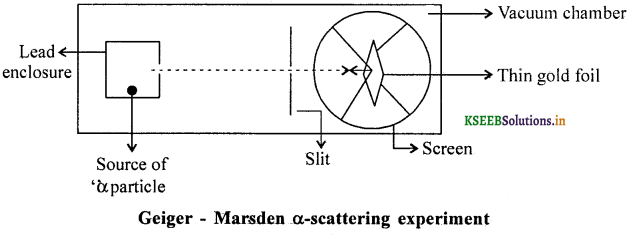The source of α – particles are taken from α – decay of 21483Bi. Gold foil used is of thickness
0.21 micron. The scattered α – particles were discovered by rotatable detector with a fluorescent flashes on the screen. The intensity of α – particles is studied as the function of ‘θ’
According the law of conservation of energy
K.E. = P.E.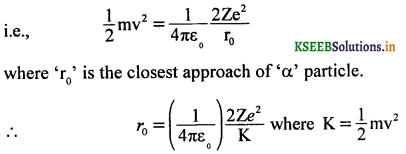Experimental conclusions:
The impact parameter Ip = 2r0 cot (θ/2).
For a wide range of values of θ, a particles travel straight because of small deflection. For θ = 180°, Ip = 0, the α – particle when directed towards the centre (for a head – on collision), it retraces the path. Thus the impact parameter is inversely proportional to tan (θ/2).Question 9.
Give the experimental conclusions arrived by Rutherford in the α – scattering experiment or State the postulates of Rutherford atom model.
Conclusions (postulates) :

1. The entire mass of the atom is concentrated in the nucleus of an atom.
2. The entire charge is concentrated in the nucleus rather than being distributed through out the volume of the atom.
3. The size of the nucleus is estimated to be of the order of 10~15 m and that of the atom of the order 10_10m.
4. The size of the electron is negligibly small and the space between the electron and nucleus, is almost void.
5. Atom as a whole is electrically neutral.
6. Electron is acted upon by a force and hence it is not in a state of static equilibrium.
7. To explain the H,- spectrum, Rutherford proposed radiating circular orbits, and for stability, he assumed that the centripetal force is balanced by the electrostatic force of attraction.Question 10.
Using Balmer empirical formula, obtain the wavelengths of Hα, Hβ, Hγ, Hσ, ……….. H### 2nd PUC Physics Atoms List of Formulae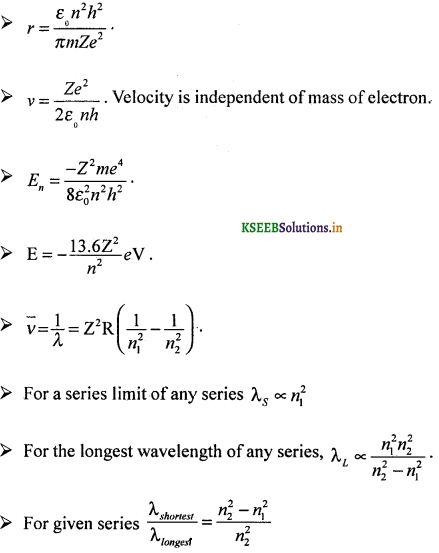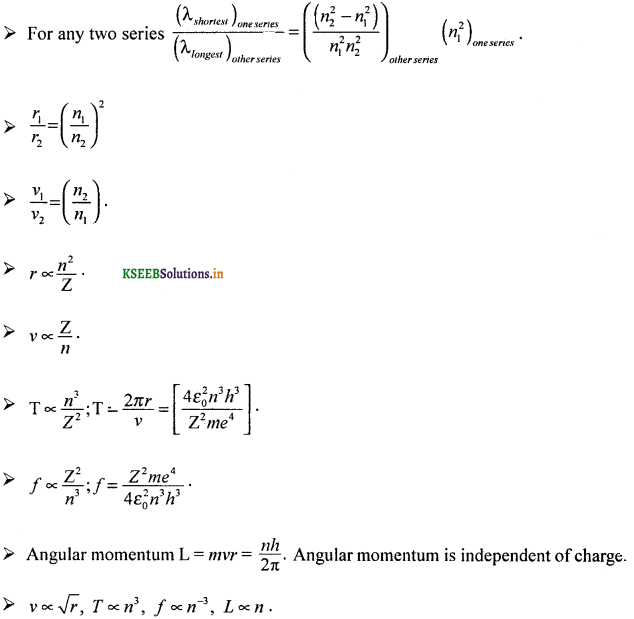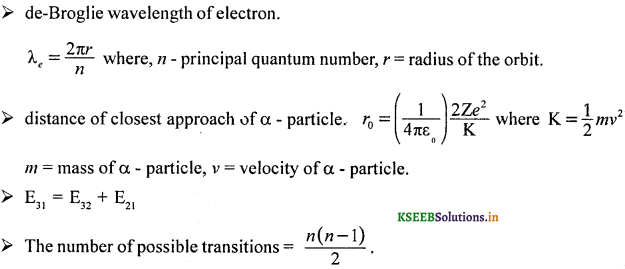### 2nd PUC Physics Atoms Numericals and Solutions

Question 1.
Calculate the radius and velocity of the electron in the nth orbit in hydrogen atom from the following data, e = 1.6 × 10-19. C, m = 9.11 × 10-31, h = 6.625 × 10-34 JS, ε0 = 8.85 × 10-12 Fm-1 and c = 3 × 10s ms-1.
Given e = 1.6 × 10-19. C, m = 9.11 × 10-31kg, h = 6.625 × 10-34 JS, ε0 = 8.85 × 10-12 Fm-1 and c = 3 × 10s ms-1.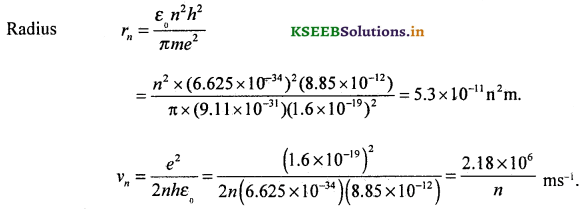Question 2.
Calculate the wavelength of Hα line (first member of Balmer series) and also Balmer series limit. Given R = 1.097 × 107 m-1.Question 3.
Calculate K.E., P.E and total energy of the electron in Bohr’s first orbit of an hydrogen atom.
Given n = 1, Assume other constants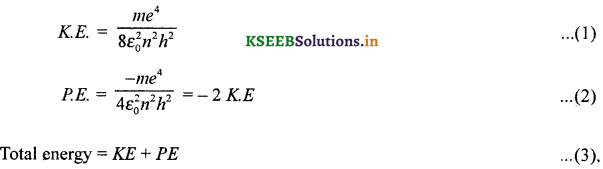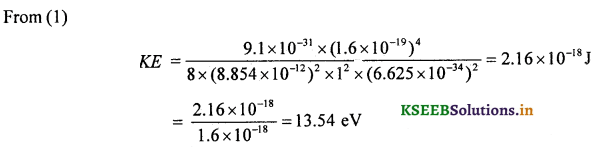From (2) PE = -2 × 13.54 eV = -27.08 eV
From (3) TE = KE + PE= 13.54 + (-27.08) = – 13,54 eV.Question 4.
Calculate the value of Rydberg’s constant given m = 9.1 × 10-31 kg, e = 1.6 × 10-19 C, ε0 = 8.854 × 10-12 Fm-1, c = 3 × 108 ms1 , h = 6.625 × 10-34 Js.
Rydberg’s constant,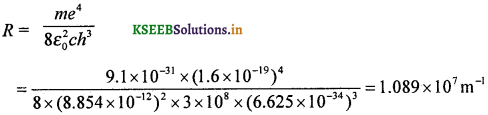Question 5.
Calculate the velocity of the electron in the first orbit of hydrogen atom. Compare this speed with the speed of light.
Given n = 1, Assume the necessary data y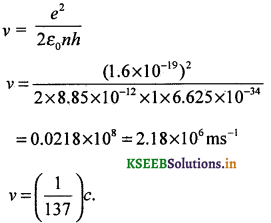Question 6.
Calculate the longest wavelength in Balmer series and the series limit. (Given R = 1.097 × 107 m-1).
For Balmer series,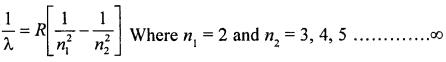(i) Longest wavelength in Balmer series corresponds to that of H2 line for which n2 = 3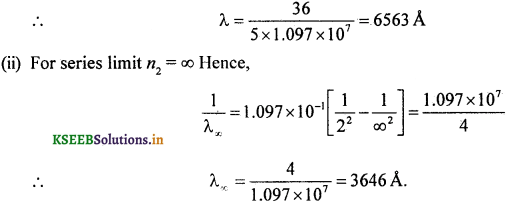Question 7.
Calculate the frequency of revolution of the electron in the ground state of hydrogen atom.
Let V be the velocity of the electron in the ground state of hydrogen atom and the radius of first orbit. We have,Question 8.
Calculate the wave number, wavelength and frequency of the spectral line of hydrogen for the transition from n2 = 4 to n1 = 2. (Given R = 1.097 × 107 m-1).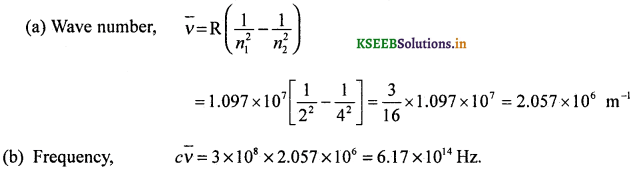Question 9.
When a certain energy is applied to an hydrogen atom, an electron jumps from n = 1 to n = 3 state. Find
(i) the energy absorbed by the electron,
(ii) Wavelength of radiation emitted when the electron jumps back to its initial state. (Energy of electron in first orbit = – 13.6 eV ; Planck’s constant – 6.6225 × 10-34 Js; Charge on electron = 1.6 × 10-19 C; Speed of light in vacuum = 3 × 108 ms-1).Question 10.
The last member of Lymann series of Hydrogen atom is 912Å. Calculate the wavelength of series limit of Balmer series.
Given : λ = 912 × 10-10 m.
For Lyman series, n1 = 1, n2 = 2, 3, 4, …. ∞
For last member, the transition is from infinity to n1 = 1 state.∴ R = 1.096 × 107 m-1
For Balmer series n1 = 2, n2 = 3, 4, 5, …. ∞
For series limit, n1 = 2, n2 = ∞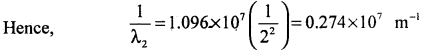Series limit, λL = $$\frac{1}{0.274 \times 10^{7}}$$
i.e., λL = 3.6496 × 10-7 m
or λL = 3649.6ÅQuestion 11.
A 12.5eV electron beam is used to bombard gaseous hydrogen at room temperature. What series of wavelengths will be emitted.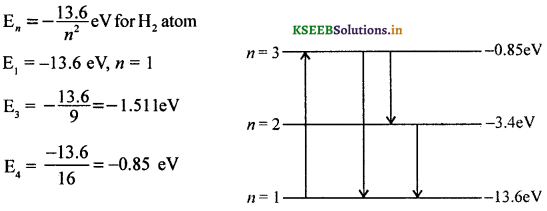So that E2 – E1 = 12.08 eV
and E4 – E1 = 12.75 eV
Since applied beam has energy <12.75eV, the excitation will be upto E3.
possible number of transitions = 3.
There will be two spectral lines in the Lyman series and one in the Balmer series.

Question 12.
In accordance with the Bohr’s model, find the quantum number that characterises Earth’s revolution around the Sun in an orbit of radius 1.5 × 1011m with orbital speed 3 × 104 ms-1 (mass of the earth = 6.0 × 1024 kg).